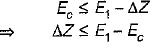Courses

# Test: Flow Through Open Channels - 3

## 20 Questions MCQ Test Topicwise Question Bank for Civil Engineering | Test: Flow Through Open Channels - 3

Description
This mock test of Test: Flow Through Open Channels - 3 for Civil Engineering (CE) helps you for every Civil Engineering (CE) entrance exam. This contains 20 Multiple Choice Questions for Civil Engineering (CE) Test: Flow Through Open Channels - 3 (mcq) to study with solutions a complete question bank. The solved questions answers in this Test: Flow Through Open Channels - 3 quiz give you a good mix of easy questions and tough questions. Civil Engineering (CE) students definitely take this Test: Flow Through Open Channels - 3 exercise for a better result in the exam. You can find other Test: Flow Through Open Channels - 3 extra questions, long questions & short questions for Civil Engineering (CE) on EduRev as well by searching above.
QUESTION: 1

### Which of the following conditions are applicable for critical flow

Solution:

For a critical flow: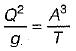Or,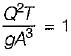QUESTION: 2

### The Chezy’s constant C in the Chezy’s equation for mean velocity in open channels is

Solution:

Chezy’s equation, V =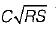Substituting dimensions of each term in above equation.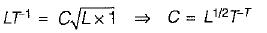QUESTION: 3

### The conveyance K of a channel is a measure of its carrying capacity. In terms of K, the discharge Q is given by

Solution:

Conveyance K = 1/n(AR2/3). It expresses the discharge capacity of the channel per unit longitudinal slope.

QUESTION: 4

A steady discharge of 1 cumec flows uniformly in a rectangular channel 1 m wide at a depth of 250 mm. The slope of the channel bed is

Solution:

Critical depth of flow :
q = Q/B = 1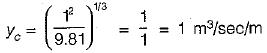⇒ Yc = 0.467
Yc = 0.5
And normal depth of flow = yn = 0.25 m
since yn < yc therefore channel has steep slope.

QUESTION: 5

In case of a hydraulic jump in a rectangular channel, the post jump, depth d2, when Froude number F1 is √3 , is
where d1 = pre-jump depth

Solution: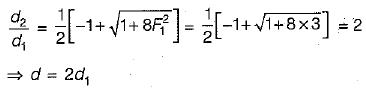QUESTION: 6

The flow characteristic before and after a hydraulic jump are such that:

Solution:

The loss of energy in a hydraulic jump leads to unequal specific energies at downstream and upstream sections.

QUESTION: 7

The figure below shows a gradually varied flow situation in an open channel with a break in bed slope. Types of water surface profiles occurring from left to right are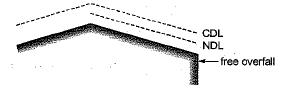Solution:
QUESTION: 8

The sequent depth ratio in a. hydraulic jump formed in a horizontal rectangular channel is 16.48. The Froude number of the supercritical stream is

Solution: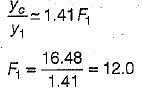QUESTION: 9

An open channel carries water with a velocity of 0.605 m/s. If the average bed shear stress is 1.0 N/m2, then the Chezy’s constant C is equal to

Solution:

As we know,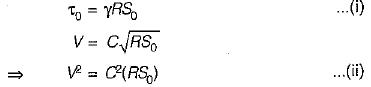Dividing (i) by (ii) we get,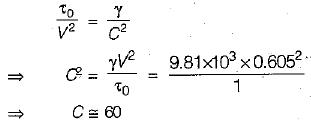QUESTION: 10

The Chezy’s and Manning’s formulae are related by

Solution:

The Manning’s n, Chezy’s C and Darcy-Weisbach’s 'f' are related as,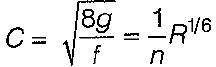QUESTION: 11

Open channel flow is the one in which

Solution:

Flow in a conduit with a free surface is known as open channel flow, flows in irrigation channels, streams and rivers, navigation channels, drainage channels and severs are some examples of open channel flow.

QUESTION: 12

In a subdritica! flow, as the specific energy in a channel is decreased, the depth of flow

Solution:
QUESTION: 13

If the specific energy at the upstream section of a rectangular channel is 3 m and minimum specific energy is 2.5 m, the maximum height of jump without causing afflux will be

Solution:
QUESTION: 14

If the Froude’s number before a hydraulic jump occurring in a rectangular horizontal channel is √10 then the Froude’s number after the jump will be

Solution: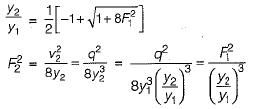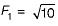Or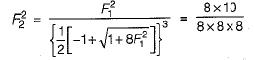∴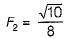QUESTION: 15

Least possible value of correction factor for
1. kinetic energy is zero
2. kinetic energy is 1
3. momentum is zero
4. momentum is 1
The correct statements are

Solution:
QUESTION: 16

If velocity is zero over 1/3rd of a cross-section and is uniform over remaining 2/3rd of the cross- section, then the correction factor for kinetic energy is

Solution:
QUESTION: 17

The depth of flow for maximum velocity in a circular channel section with diameter equal to 1.5 m is

Solution:
QUESTION: 18

The critical depth of flow in a most economical triangular channel section for a discharge of 1 m3/sec is given by

Solution:
QUESTION: 19

The mild slope profile M2 ocdufs for depth

Solution:
QUESTION: 20

Gradually varied flow in open channel is caused when

Solution:

If the flow is subcritical, then after the introduction of hump the specific energy will decrease by ΔZ (height of hump).